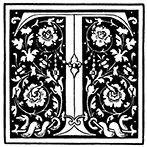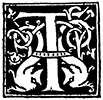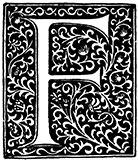﻿ Newton's Second Law | Zona Land Education

# Ways To Say Newton's Second Law

Here is a collection of statements of Isaac Newton's second law of motion in words similar to those often presented in high school and college textbooks. Click the 'More' button near each statement, and the page will expand with an explanation.

Basically, the second law describes how the acceleration of an object relates to the applied net force on the object, and how the acceleration of an object relates to the mass of the object. Large forces make for large accelerations; small forces make for small accelerations. Large masses make for small accelerations, small forces make for large accelerations.he effect of an applied force is to cause the body to accelerate in the direction of the force. The acceleration is in direct proportion to the force and in inverse proportion to the mass of the body.

 'The effect of an applied force' When the net force on the object is not zero. 'cause the body to accelerate in the direction of the force' The acceleration vector points in the same direction as the net force vector. 'The acceleration is in direct proportion to the force' With the mass constant, by whatever factor the force changes, the acceleration changes by that same factor. The acceleration is ... in inverse proportion to the mass' With the net force constant, by whatever factor the mass changes, the acceleration changes by the reciprocal, or inverse, of that factor.o give a mass m an acceleration a, a net force given by F = ma is required.

 'To give a mass m an acceleration a' Use the variable 'm' for mass, 'a' for acceleration. This is about an accelerating object; that is, the object's velocity is not constant. 'a net force given by F = ma is required' Use the variable 'F' for net force. This net force is non-zero. Basically, if you want to accelerate the object, you need to apply a non-zero net force. That non-zero net force can be calculated by multiplying the mass of the object times its acceleration. This formula contains the proportions described in the first statement above.

Note that as stated here the formula F = ma has bold letters for force and acceleration. These bold letters mean that these quantities are vectors. The mass, with the non-bold letter is a scalar. Multiplication of the acceleration vector by the mass scalar will not change the direction of the calculation. Therefore, the force vector, which is the result of the multiplication, has the same direction as the acceleration vector. This is implied in this notation.he acceleration of an object is directly proportional to the net force acting on it and is inversely proportional to its mass. The direction of the acceleration is in the direction of the net force acting on the object.

 'The acceleration of an object is directly proportional to the net force acting on it' By whatever factor the net force changes, the acceleration changes by that same factor. 'The acceleration ... is inversely proportional to its mass' By whatever factor the mass changes, the acceleration changes by the reciprocal, or inverse, of that factor. 'The direction of the acceleration is in the direction of the net force' The direction of the acceleration vector is the same as the direction of the net force vector.he acceleration of an object is directly proportional to the net external force acting on the object and inversely proportional to the mass of the object.

 'The acceleration of an object is directly proportional to the net external force' By whatever factor the net force changes, the acceleration changes by the same factor. 'The acceleration ... is ... inversely proportional to the mass' By whatever factor the mass changes, the acceleration changes by the reciprocal, or inverse, of that factor.

Note that this statement does not say that the net force vector and the acceleration vector point in the same direction, which they do.= ma

The formula F = ma is often said to be Newton's second law, and that's usually considered correct. However, a complete understanding includes seeing that this equation, written in vector form, contains all the information written in the first statement on this page.ate of change of momentum is proportional to the impressed force, and is in the direction in which the force acts.

These are close to Newton's own words when he expressed his second law of motion. Newton described this law in terms of momentum much as this statement does.

 'Rate of change of momentum' This is the time rate change in momentum. It is delta p divided by delta t. It is the change in momentum per time unit. 'proportional' This is a direct proportion. By whatever factor the rate change in momentum changes, the impressed force changes by the same factor. By whatever factor delta p divided by delta t changes, the impressed force changes by the same factor. As we will see below, the rate of change of momentum literally is the impressed force, so, of course, they are directly proportional. 'impressed force' This is the net force, or the total force. 'in the direction in which the force acts' The direction in which the rate of change of momentum changes is aimed the same as the impressed force. As we will see below, the rate of change in momentum literally is the impressed force, so, of course, they are in the same direction.

Here is a derivation that shows how this all works out:

Custom Search